NCERT Solutions: Knowing Our Numbers

# NCERT Solutions: Knowing Our Numbers - Notes | Study Mathematics (Maths) Class 6 - Class 6

 1 Crore+ students have signed up on EduRev. Have you?

Exercise 1.1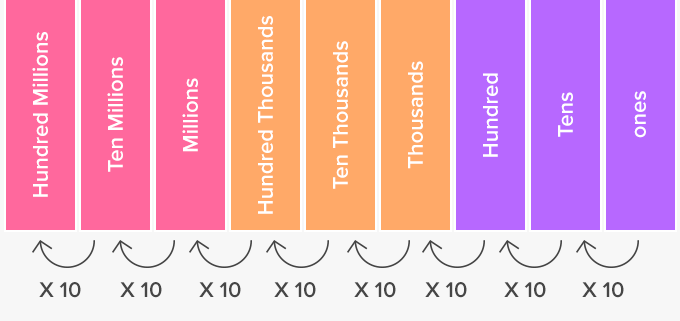Place Value ChartQ1. Fill in the blanks:
(a) 1 lakh = ______ten thousand
(b) 1 million = ______hundred thousand
(c) 1 crore = ______ten lakh
(d) 1 crore = ______million
(e) 1 million = ______lakh
Ans:
(a) 10

• Since ten thousand contains 4 zeroes and one lakh has 5, multiplying ten by ten thousand will equal one lakh.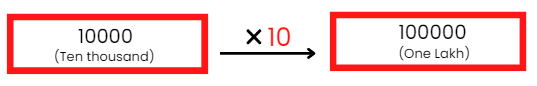Hence, 1 lakh = 10 ten thousand

(b) 10

• Since a hundred thousand contains 5 zeroes and one million has 6, multiplying ten by a hundred thousand will equal 1 million.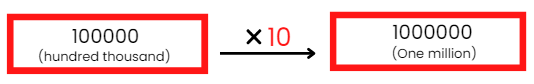Hence, 1 million = 10 hundred thousand

(c) 10

• Since ten lakh contains six zeroes and one crore has seven, multiplying ten by ten lakh will equal one crore.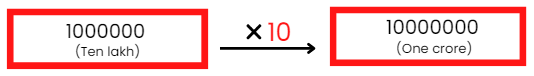Hence, 1 crore = 10 ten lakh

(d) 10

• Since 1 million contains six zeroes and one crore has seven, multiplying ten by 1 million will equal 1 crore.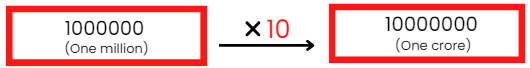Hence, 1 crore = 10 million

(e) 10

• Since 1 lakh contains 5 zeroes and one million has six, multiplying ten by 1 lakh will equal 1 million.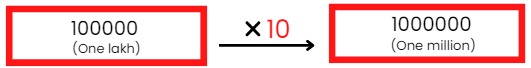Hence, 1 million = 10 lakh

Q2. Place commas correctly and write the numerals:
(a) Seventy-three lakh seventy-five thousand three hundred seven.
(b) Nine crore five lakh forty-one.
(c) Seven crore fifty-two lakh twenty-one thousand three hundred two.
(d) Fifty-eight million four hundred twenty-three thousand two hundred two.
(e) Twenty-three lakh thirty thousand ten.
Ans:

(a) 73,75,307
(b) 9,05,00,041
(c) 7,52,21,302
(d) 58,423,202
(e) 23,30,010

Q3. Insert commas suitable and write the names according to Indian system of numeration:
(a) 87595762
(b) 8546283
(c) 99900046
(d) 98432701
Ans: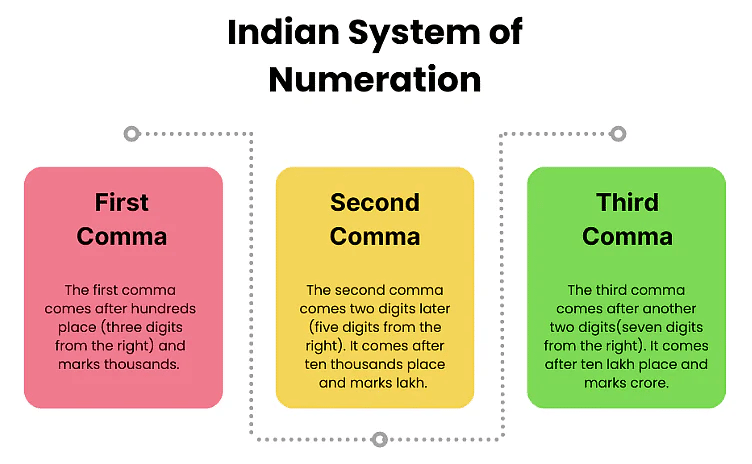(a) 8,75,95,762: Eight crore seventy-five lakh ninety-five thousand seven hundred sixty-two.
(b) 85,46,283: Eight-five lakh forty-six thousand two hundred eighty-three.
(c) 9,99,00,046: Nine crore ninety-nine lakh forty-six.
(d) 9,84,32,701: Nine crore eighty-four lakh thirty-two thousand seven hundred one.

Q4. Insert commas suitable and write the names according to International system of numeration:
(a) 78921092
(b) 7452283
(c) 99985102
(d) 48049831
Ans: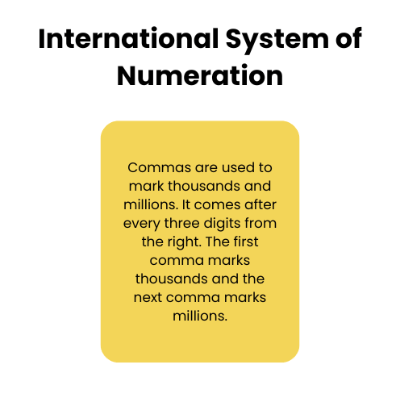(a) 78,921,092: Seventy-eight million nine hundred twenty-one thousand ninety-two.
(b) 7,452,483: Seven million four hundred fifty-two thousand two hundred eighty-three.
(c) 99,985,102: Ninety-nine million nine hundred eighty-five thousand one hundred two.
(d) 48,049,831: Forty-eight million forty-nine thousand eight hundred thirty-one.

Exercise 1.2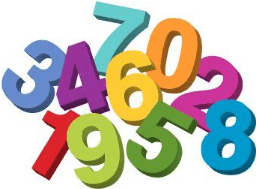Q1. A book exhibition was held for four days in a school. The number of tickets sold at the counter on the first, second, third and final day was respectively 1094, 1812, 2050 and 2751. Find the total number of tickets sold on all the four days.
Ans: Number of tickets sold on first day = 1,094
⇒ Number of tickets sold on second day = 1,812
⇒ Number of tickets sold on third day = 2,050
⇒ Number of tickets sold on fourth day = 2,751
⇒ Total tickets sold = 1,094 + 1,812 + 2,050 + 2,751 = 7,707
Therefore, 7,707 tickets were sold on all the four days.

Q2. Shekhar is a famous cricket player. He has so far scored 6980 runs in test matches. He wishes to complete 10,000 runs. How many more runs does he need?
Ans: Total runs scored by Shekhar = 6,980
Number of run he wishes to complete = 10,000
Number of runs he needs
= Total runs he wishes to get - Runs Scored
= 10,000 - 6,980
= 3020

Q3. In an election, the successful candidate registered 5,77,500 votes and his nearest rival secured 3,48,700 votes. By what margin did the successful candidate win the election?
Ans: Number of votes secured by successful candidates = 5,77,500
Number of votes secured by his nearest rival = 3,48,700
Margin by which candidate wins
= 5,77,500 - 3, 48,700 = 2,28,800

Q4. Kirti Bookstore sold books worth Rs 2,85,891 in the first week of June and books worth Rs 4,00,768 in the second week of the month. How much was the sale for the two weeks together? In which week was the sale greater and by how much?
Ans: Books sold in first week = 2,85,891
⇒ Books sold in second week = 4,00,768
⇒ Total books sold = 6,86,659
Since 4,00,768,> 2,85,891
Therefore, Sale of the second week is greater than that of the first week.
⇒ Books sold in second week = 4,00,768
⇒ Books sold in first week = 2,85,891
Difference in sales of week 1 and week 2 = 4,00,768 - 2,85,891
= 1,14,877

Q5. Find the difference between the greatest and the least number that can be written using the digits 6, 2, 7, 4, 3 each only once.
Ans: Greatest five-digit number using digits 6,2,7,4,3 = 76432
⇒ Smallest five-digit number using digits 6,2,7,4,3 = 23467
⇒ Difference = 52965
Therefore, the difference is 52965.

Q6. A machine, on average, manufactures 2,825 screws a day. How many screws did it produce in the month of January 2006?
Ans: Number of screws manufactured in one day = 2,825
⇒ Number of days in the month of January (31 days) = 2,825 x 31 = 87,575
Therefore, the machine produced 87,575 screws in the month of January.

Q7. A merchant had Rs 78,592 with her. She placed an order for purchasing 40 radio sets at Rs 1,200 each. How much money will remain with her after the purchase?
Ans: Cost of one radio = Rs 1200
⇒ Cost of 40 radios = 1200 x 40 = Rs 48,000
⇒ Now, Total money with merchant = Rs 78,592
⇒ Money spent by her = Rs 48,000
⇒ Money left with her = Rs 30,592
Therefore, Rs 30,592 will remain with her after the purchase.

Q8. A student multiplied 7236 by 65 instead of multiplying by 56. By how much was his answer greater than the correct answer?
Ans: Wrong answer = 7236 x 65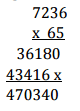Correct answer = 7236 x 56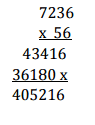Difference in answers = 470340 – 405216 = 65,124.

To Solve the questions given below, refer to this table: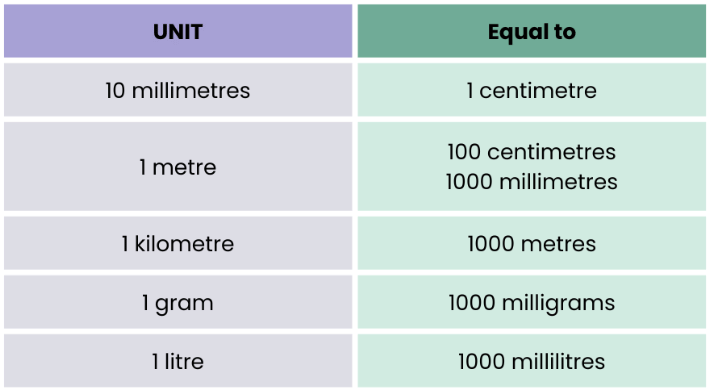Q9. To stitch a shirt 2 m 15 cm cloth is needed. Out of 40 m cloth, how many shirts can be stitched and how much cloth will remain?
Ans: Cloth required to stitch one shirt = 2 m 15 cm = (2 x 100) cm + 15 cm = 215 cm
⇒ Length of cloth = 40 m = 40 x 100 cm = 4000 cm
⇒ Number of shirts that can be stitched = 4000/215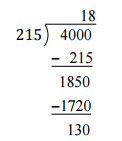Therefore, 18 shirts can be stitched and 130 cm (1 m 30 cm) cloth will remain.

Q10. Medicine is packed in boxes, each weighing 4 kg 500 g. How many such boxes can be loaded in a can which cannot carry beyond 800 kg?
Ans: The weight of one box = 4 kg 500 g = 4 x 1000 g + 500 g = 4500 g
⇒ Maximum load can be loaded in van = 800 kg = 800 x 1000 g = 800000 g
⇒ Number of boxes = 800000/4500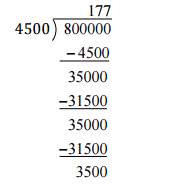Therefore, 177 boxes can be loaded.

Q11. The distance between the school and the house of a student’s house is 1 km 875 m. Every day she walks both ways. Find the total distance covered by her in six days.
Ans: Distance between school and home = 1.875 km
⇒ Distance between home and school = + 1.875 km
⇒ Total distance covered in one day = 3.750 km
⇒ Distance covered in six days = 3.750 x 6 = 22.500 km.
Therefore, 22 km 500 m distance covered in six days.

Q12. A vessel has 4 liters and 500 ml of curd. In how many glasses each of 25 ml capacity, can it be filled?
Ans: Capacity of curd in a vessel = 4 litres 500 ml = 4 x 1000 ml + 500 ml = 4500 ml
⇒ Capacity of one glass = 25 ml
⇒ Number of glasses can be filled = 4500/25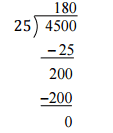Therefore, 180 glasses can be filled by curd.

Exercise 1.3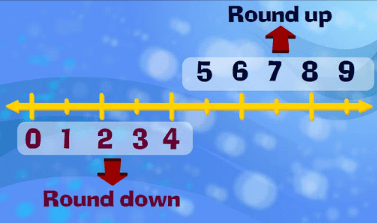Q1. Estimate each of the following using general rule:
(a) 730 + 998
(b) 796 – 314
(c) 12,904 + 2,888
(d) 28,292 – 21,496
Ans:
(a) 730 round off to 700

⇒ 998 round off to 1000
⇒ Estimated sum = 1700
(b) 796 round off to 800
⇒ 314 round off to 300
⇒ Estimated sum = 500
(c) 12904 round off to 13000
⇒ 2888 round off to 3000
⇒ Estimated sum = 16000
(d) 28292 round off to 28000
⇒ 21496 round off to 21000
⇒ Estimated difference = 7000

Q2. Give a rough estimate (by rounding off to nearest hundreds) and also a closer estimate (by rounding off to nearest tens):
(a) 439 + 334 + 4317
(b) 1,08,737 – 47,599
(c) 8325 – 491
(d) 4,89,348 – 48,365
Ans:
(a) 439 round off to 400
⇒ 334 round off to 300
⇒ 4317 round off to 4300
⇒ Estimated sum = 5000
(b) 108737 round off to 108700
⇒ 47599 round off to 47600
⇒ Estimated difference = 61100
(c) 8325 round off to 8300
⇒ 491 round off to 500
⇒ Estimated difference = 7800
(d) 489348 round off to 489300
⇒ 48365 round off to 48400
⇒ Estimated difference = 440900

Q3. Estimate the following products using general rule:
(a) 578 x 161
(b) 5281 x 3491
(c) 1291 x 592
(d) 9250 x 29
Ans:
(a) 578 x 161
⇒ 578 round off to 600
⇒ 161 round off to 200
⇒ The estimated product = 600 x 200 = 1,20,000
(b) 5281 x 3491
⇒ 5281 round of to 5,000
⇒ 3491 round off to 3,500
⇒ The estimated product = 5,000 x 3,500 = 1,75,00,000
(c) 1291 x 592
⇒ 1291 round off to 1300
⇒ 592 round off to 600
⇒ The estimated product = 1300 x 600 = 7,80,000
(d) 9250 x 29
⇒ 9250 round off to 9,000
⇒ 29 round off to 30
⇒ The estimated product = 9,000 x 30 = 2,70,000

The document NCERT Solutions: Knowing Our Numbers - Notes | Study Mathematics (Maths) Class 6 - Class 6 is a part of the Class 6 Course Mathematics (Maths) Class 6.
All you need of Class 6 at this link: Class 6

## Mathematics (Maths) Class 6

168 videos|282 docs|43 tests

## Mathematics (Maths) Class 6

168 videos|282 docs|43 tests

Track your progress, build streaks, highlight & save important lessons and more!

,

,

,

,

,

,

,

,

,

,

,

,

,

,

,

,

,

,

,

,

,

;